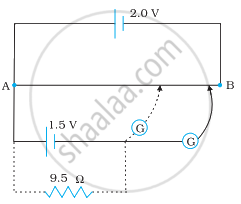# Figure shows a 2.0 V potentiometer used for the determination of internal resistance of a 1.5 V cell. The balance point of the cell in open circuit is 76.3 cm. - Physics

Numerical

Figure shows a 2.0 V potentiometer used for the determination of internal resistance of a 1.5 V cell. The balance point of the cell in open circuit is 76.3 cm. When a resistor of 9.5 Ω is used in the external circuit of the cell, the balance point shifts to 64.8 cm length of the potentiometer wire. Determine the internal resistance of the cell.#### Solution

Internal resistance of the cell = r

Balance point of the cell in open circuit, l1 = 76.3 cm

An external resistance (R) is connected to the circuit with R = 9.5 Ω

New balance point of the circuit, l= 64.8 cm

Current flowing through the circuit = I

The relation connecting resistance and emf is,

r = (("l"_1 - "l"_2)/"l"_2) "R"

= (76.3 - 64.8)/64.8 xx 9.5

= 1.68 Ω

Therefore, the internal resistance of the cell is 1.68 Ω.

Concept: Potentiometer
Is there an error in this question or solution?

#### APPEARS IN

NCERT Physics Part 1 and 2 Class 12
Chapter 3 Current Electricity
Exercise | Q 3.23 | Page 131
NCERT Class 12 Physics Textbook
Chapter 3 Current Electricity
Exercise | Q 23 | Page 130
Share